### Home > INT3 > Chapter Ch12 > Lesson 12.1.2 > Problem12-24

12-24.

Jeremy uses the $\sin^{-1}$ button on his calculator to solve a trigonometric equation and gets the solution $37^\circ$. He knows that there must be more than one solution. What are the rest of the solutions to Jeremy’s equation? Use a unit circle to explain how you determined all of the solutions. Homework Help ✎

Using a unit circle:

Draw a Unit circle and estimate $37$ degrees.

Since sin is height, draw in the height to the $x$-axis.

Find and draw the same height any other place it may occur on the unit circle.

Calculate the new angles.

$180^\circ − 37^\circ = 143^\circ$, so $37 + 2πn$ and $143 + 2πn$ where $n$ is any integer.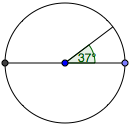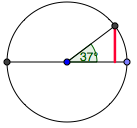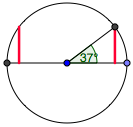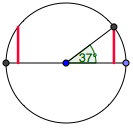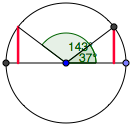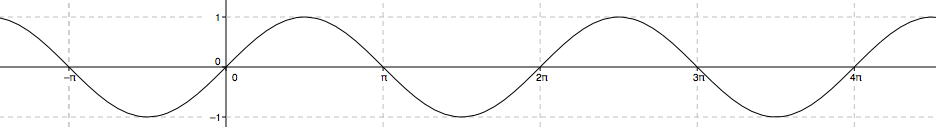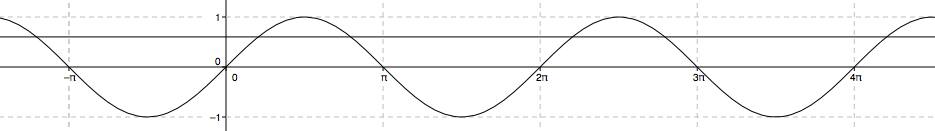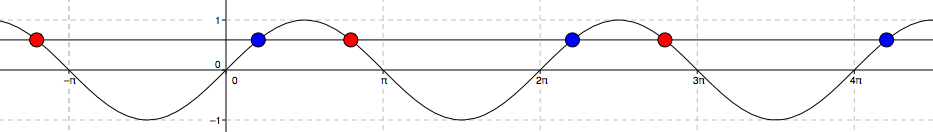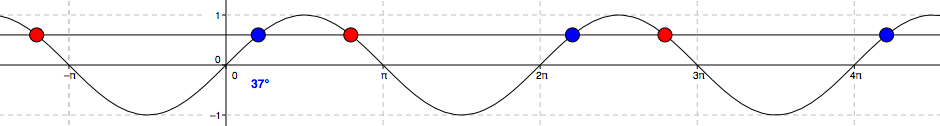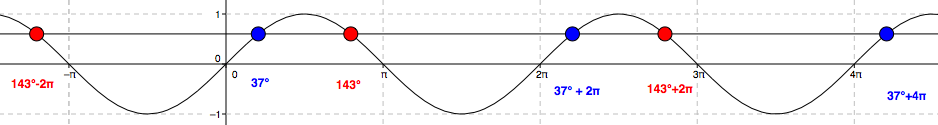Using a graph:

Draw the sine curve.

Draw $y=\sin(37)$

Find the $x$-values where the two graphs intersect.

Label the solution $37^\circ$.

Use Symmetry to find other solutions.